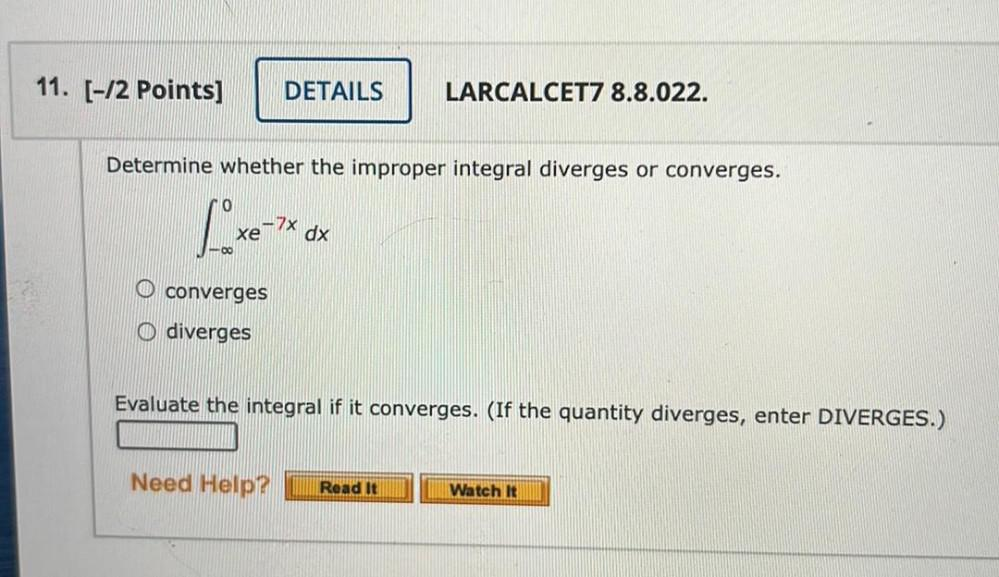Question:

# Determine whether the improper integral diverges or converges. 0 ∫ xe^(-7x).dx -∞ a) converges b) diverges Evaluate the integral if it converges. (If the quantity diverges, enter DIVERGES.)Determine whether the improper integral diverges or converges. 0 ∫ xe^(-7x).dx -∞ a) converges b) diverges Evaluate the integral if it converges. (If the quantity diverges, enter DIVERGES.)